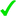# Zelda Wiki

This is the main module for the following templates: In addition, this module exports the following functions.

## sort

`sort(game, sequence, terms)`

Parameters
Returns
• The array of terms, sorted according to the given sequence for the given game, minus those that aren't in the sequence at all.
• An array of the terms that were not sorted.
Examples
InputOutputStatus
```sort(
"ST",
"Realm",
{
"Fire Realm",
"Sand Realm",
"💩Realm",
"Ocean Realm",
"Forest Realm",
"Fire Realm",
}
)
```
```{
"Forest Realm",
"Ocean Realm",
"Fire Realm",
"Sand Realm",
}
``````{"💩Realm"}
```## getSortValue

`getSortValue(game, sequence, term)`

Parameters
Returns
• Integer sort value of `term` for the given `sequence` in `game`, or `nil` if none found.
Examples
InputOutputStatus
```getSortValue("ST", "Realm", "Snow Realm")
```
```2
``````getSortValue("ST", "Realm", "💩 Realm")
```
```nil
``````local p = {}
local h = {}

local utilsArg = require("Module:UtilsArg")
local utilsCargo = require("Module:UtilsCargo")
local utilsMarkup = require("Module:UtilsMarkup")
local utilsString = require("Module:UtilsString")
local utilsTable = require("Module:UtilsTable")

local cargoTable = "Sequences"

function p.CargoStore(frame)
local args, err = utilsArg.parse(frame:getParent().args, p.Templates["Sequence/Store"])
if err then
return utilsMarkup.categories(err.categories)
end
for i, v in ipairs(args.terms) do
frame:callParserFunction({
name = "#cargo_store:_table=" .. cargoTable,
args = {
ordinality = i,
term = v,
game = mw.title.getCurrentTitle().subpageText,
sequence = args.sequence,
}
})
end
end

function p.SortValue(frame)
local args, err = utilsArg.parse(frame:getParent().args, p.Templates["Sort Value"])
if err then
return utilsMarkup.categories(err.categories)
end
local game = args.game
local sequence = args.sortBy
local term = args.term

local sortValue = p.getSortValue(game, sequence, term)
if not sortValue then
local warn = ("Sort value not found for <code>%s</code> in game <code>%s</code>. Defaulting to alphabetical. See [[Data:Sequences/%s]] for list of valid sort values.")
:format(term, game, game)
return ""
end
local dataSortValue = string.format('data-sort-value="%s"|', sortValue)
return dataSortValue
end

function p.sort(game, sequence, terms)
local queryResults = utilsCargo.query(cargoTable, "term", {
where = utilsCargo.allOf({
game = game,
sequence = sequence
}),
orderBy = "ordinality",
})
local validTerms = utilsTable.map(queryResults, "term")
local sorted = utilsTable.intersection(validTerms, terms)
local unsorted = utilsTable.difference(terms, sorted)
return sorted, unsorted
end

function p.getSortValue(game, sequence, term)
local fields = "term, sequence, ordinality"
local queryResults = utilsCargo.query(cargoTable, fields, {
where = utilsCargo.allOf({
game = game,
term = term,
sequence = sequence,
}),
orderBy = "ordinality",
})
local sortValue = queryResults and tonumber(queryResults.ordinality)
return sortValue
end

local game = {
type = "string",
required = true,
desc = "A string representing a game or other published title.",
}

local sequence = {
type = "string",
required = true,
desc = "A string identifiying a sequence.",
}

p.Schemas = {
sort = {
game = game,
sequence = sequence,
terms = {
type = "array",
required = true,
items = { type = "string" },
desc = "An array of strings to sort according to the given sequence.",
}
},
getSortValue = {
game = game,
sequence = sequence,
term = {
type = "string",
required = true,
desc = "Term to get sort value for.",
}
}
}

p.Templates = {
["Sequence/Store"] = {
purpose = "Stores data regarding in-game sequences (e.g. trading sequences, dungeon orders, boss orders, etc.), which can then be used for sorting purposes (see [[Template:Sort Value]]).",
storesData = "Data:Sequences",
params = {
 = {
name = "sequence",
type = "string",
required = true,
desc = "An identifier name for to the sequence.",
trim = true,
inline = true,
},
["..."] = {
name = "terms",
placeholder = "term",
type = "string",
desc = "Sequence terms.",
trim = true,
},
},
format = "block",
examples = {
{
desc = "Example from [[Data:Sequences/ST]]",
args = {"Realm", "Forest Realm", "Snow Realm", "Ocean Realm", "Fire Realm", "Sand Realm"}
}
}
},
["Sort Value"] = {
purpose = "Displaying [[Guidelines:Terminology|term]] links in [[Help:Tables#Sortable_Tables|sortable tables]], sorted according to some in-game sequence defined at [[Data:Sequences]].",
usesData = "Data:Sequences",
params = {
 = {
name = "game",
type = "string",
required = true,
desc = "The game or other published title to which the term applies.",
},
 = {
name = "term",
type = "string",
required = true,
desc = "The term to be sorted. Should be a valid [[Guidelines:Terminology|Term]]",
},
sortBy = {
type = "string",
canOmit = true,
desc = "If the given term belongs to more than one sequence in the given game, use this parameter to specify which sequence to use for sorting."
},
},
format = "inline",
},
}

p.Documentation = {
sort = {
params = {"game", "sequence", "terms"},
returns = {
"The array of terms, sorted according to the given sequence for the given game, minus those that aren't in the sequence at all.",
"An array of the terms that were not sorted."
},
cases = {
outputOnly = true,
{
args = {"ST", "Realm", {"Fire Realm", "Sand Realm", "💩Realm", "Ocean Realm", "Forest Realm", "Fire Realm" }},
expect = {
{ "Forest Realm", "Ocean Realm", "Fire Realm", "Sand Realm" },
{ "💩Realm" },
},
},
},
},
getSortValue = {
params = {"game", "sequence", "term"},
returns = "Integer sort value of <code>term</code> for the given <code>sequence</code> in <code>game</code>, or <code>nil</code> if none found.",
cases = {
outputOnly = true,
{
args = {"ST", "Realm", "Snow Realm"},
expect = 2
},
{
args = {"ST", "Realm", "💩 Realm"},
expect = nil
},
}
},
}

return p
```